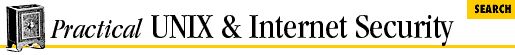#Chapter 23Writing Secure SUID and Network Programs## 23.7 UNIX Pseudo-Random Functions

The standard UNIX C library provides two random number generators: rand( ) and random( ). A third random number generator, drand48( ), is available on some versions of UNIX. Although you won't want to use any of these routines to produce cryptographic random numbers, we'll briefly explain each. Then, if you need to use one of them for something else, you'll know something about its strengths and shortcomings.

### 23.7.1 rand ( )

The original UNIX random number generator, rand( ), is not a very good random number generator. It uses a 32-bit seed and maintains a 32-bit internal state. The output of the function is also 32 bits in length, making it a simple matter to determine the function's internal state by examining the output. As a result, rand( ) is not very random. Furthermore, the low-order bits of some implementations are not random at all, but flip back and forth between 0 and 1 according to a regular pattern. The rand( ) random number generator is seeded with the function srand( ). On some versions of UNIX, a third function is provided, rand_r( ), for multi threaded applications. (The function rand( ) itself is not safe for multi- threading, as it maintains internal state.)

Do not use rand( ), even for simple statistical purposes.

### 23.7.2 random ( )

The function random( ) is a more sophisticated random number generator which uses nonlinear feedback and an internal table that is 124 bytes (992 bits) long. The function returns random values that are 32 bits in length. All of the bits generated by random( ) are usable.

The random( ) function is adequate for simulations and games, but should not be used for security related applications such as picking cryptographic keys or simulating one- time pads.

### 23.7.3 drand48 ( ), lrand48 ( ), and mrand48 ( )

The function drand48( ) is one of many functions which make up the System V random number generator. According to the Solaris documen- tation, the algorithm uses "the well-known linear congruential algorithm and 48-bit integer arithmetic." The function drand48( ) returns a double-precision number that is greater or equal to 0.0 and less than 1.0, while the lrand48( ) and mrand48( ) functions return random numbers within a specified integer range. As with random( ), these functions provide excellent random numbers for simulations and games, but should not be used for security-related applications such as picking cryptographic keys or simulating one-time pads; linear congruential algorithms are too easy to break.

### 23.7.4 Other random number generators

There are many other random number generators. Some of them are optimized for speed, while others are optimized for randomness. You can find a list of other random number generators in Bruce Schneier's excellent book, Applied Cryptography (John Wiley & Sons, Second Edition, 1995).

Some versions of the Linux operating system have carefully thought out random number generators in their kernel, accessible through the /dev/random and /dev/urandom devices. We think that this design is excellent-especially when the random number generators take into account additional system states, user inputs, and "random" external events to provide numbers that are "more" random.23.6 Tips on Generating Random Numbers23.8 Picking a Random Seed